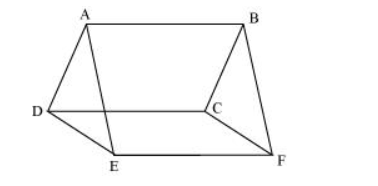# In the following figure, ABCD, DCFE and ABFE are parallelograms.Question. In the following figure, ABCD, DCFE and ABFE are parallelograms. Show that ar (ADE) = ar (BCF).Solution:

It is given that ABCD is a parallelogram. We know that opposite sides of a parallelogram are equal.

$\therefore A D=B C \ldots(1)$

Similarly, for parallelograms DCEF and ABFE, it can be proved that

$D E=C F \ldots(2)$

And, $E A=F B \ldots(3)$

In $\triangle \mathrm{ADE}$ and $\triangle B C F$,

$A D=B C$ [Using equation (1)]

$D E=C F[U \sin g$ equation $(2)]$

$E A=F B$ [Using equation (3)]

$\therefore \triangle \mathrm{ADE} \cong \mathrm{BCF}($ SSS congruence rule $)$

$\Rightarrow$ Area $(\triangle A D E)=$ Area $(\triangle B C F)$